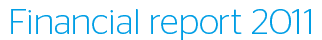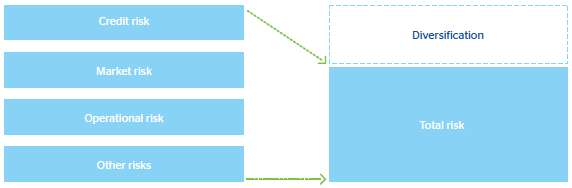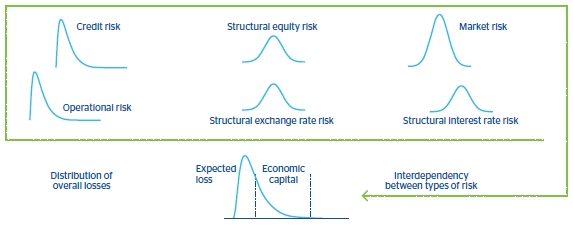# Integration of risks

The economic capital required to cover the Group’s losses is calculated by integrating the various risks managed by the Institution. The difference between this required economic capital and the sum of the individual capital amounts is known as the benefit of diversification.

BBVA Group’s risk integration model recognizes diversification among the various types of risks. The calculation process is divided into two stages. In the first stage, each of the risks is modeled individually (credit, market, structural and operational), taking the special features of each case into account. In the second stage, they are added to a common measurement through a model that looks at the structure of dependency between risks.

In this framework, the diversification level of each risk depends mainly, on the relative size of the risk against global risk, as well as on the correlation among risks and the characteristics of individual loss distributions.

###### 1. Sum of risks and diversification###### 2. Risk integration diagramTools